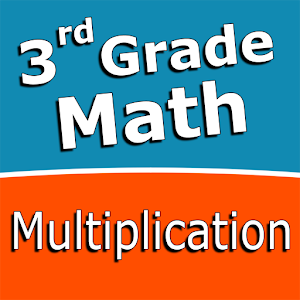Advertisement

# 24 hours or less GiveawayGOTD - Third grade Math - Multiplication

#### DownloadHR

##### Software guruRating: 3/5

Intuitive interface powered by handwriting input and a fun and engaging mini game in addition to a regular math trainer mode make our app stand out from the crowd of generic math learning apps.

With Third Grade Math - Multiplication you can practice and improve the following math skills:
• Multiplication tables for 2, 3, 4, 5, 10
• Multiplication tables for 6, 7, 8, 9
• Multiplication tables up to 10×10
• Multiplication tables up to 12×12
• Multiply by a multiple of ten
• Multiply one-digit numbers by two-digit numbers
• Multiply one-digit numbers by three-digit numbers
• Multiply three 1-digit numbers
• Multiply numbers ending in zeroes
Choose between two different modes:
• Math Trainer
• Math Shot mini-game
Math Trainer mode helps you to master the math facts, you can solve math problems at you own pace without any time constrain.

Math Shot is a math mini-game with fun and engaging game-play and as we all know learning through play and fun is more effective. The game difficulty adapts to player's skills and makes the game suitable for all ages. Math Shot helps students to develop math fact fluency - the result of consistent learning, repetition, and practice.

Giveaway page: read more

Advertisement